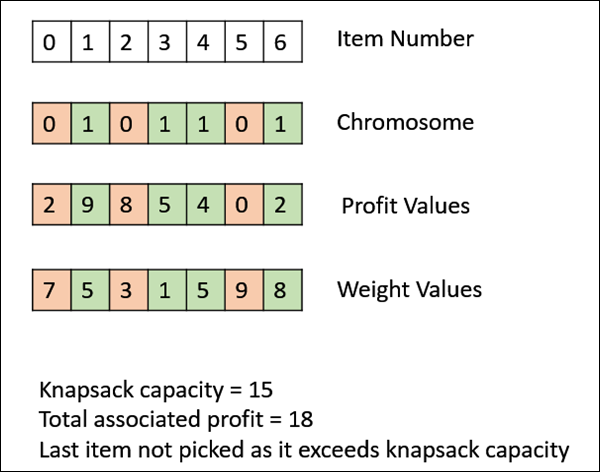Genetic Algorithms - Fitness Function

The fitness function simply defined is a function which takes a candidate solution to the problem as input and produces as output how “fit” our how “good” the solution is with respect to the problem in consideration.

Calculation of fitness value is done repeatedly in a GA and therefore it should be sufficiently fast. A slow computation of the fitness value can adversely affect a GA and make it exceptionally slow.

In most cases the fitness function and the objective function are the same as the objective is to either maximize or minimize the given objective function. However, for more complex problems with multiple objectives and constraints, an Algorithm Designer might choose to have a different fitness function.

A fitness function should possess the following characteristics −

• The fitness function should be sufficiently fast to compute.

• It must quantitatively measure how fit a given solution is or how fit individuals can be produced from the given solution.

In some cases, calculating the fitness function directly might not be possible due to the inherent complexities of the problem at hand. In such cases, we do fitness approximation to suit our needs.

The following image shows the fitness calculation for a solution of the 0/1 Knapsack. It is a simple fitness function which just sums the profit values of the items being picked (which have a 1), scanning the elements from left to right till the knapsack is full.